Next: Surface Integrals Up: Vectors Previous: Vector Calculus

## Line Integrals

A vector field is defined as a set of vectors associated with each point in space. For instance, the velocity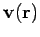in a moving liquid (e.g., a whirlpool) constitutes a vector field. By analogy, a scalar field is a set of scalars associated with each point in space. An example of a scalar field is the temperature distribution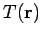in a furnace.

Consider a general vector field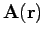. Line integrals of the form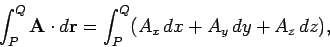(44)

evaluated on some particular path taken between two fixed points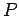and, often arise in Physics. Here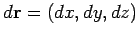is a path element. The path might be specified as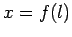,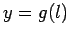, and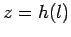, where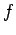,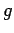,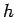are mathematical functions, and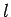is a parameter (such as path-length) which varies monotonically along the path. It follows that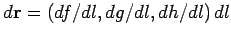. In particular, ifis a force-field then the line integral is the work done by the force in going between pointsandalong the given path [cf., Eq. (26)]. Finally, if the path is a closed loop (i.e., ifandare the same point) then the integral is conventionally written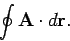(45)

As an example of a path integral, consider the work done in a repulsive, inverse-square, central field,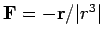. The element of work done is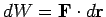. Take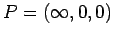and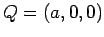. Route 1 is along the-axis, so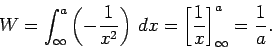(46)

The second route is, firstly, around a large circle (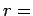constant) to the point (,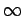, 0), and then parallel to the-axis--see Fig. 8. In the first, part no work is done, since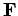is perpendicular to. In the second part,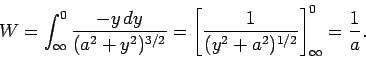(47)

In this case, the integral is independent of the path taken between the beginning and end points. However, not all line integrals are path independent. Indeed, there are two different classes of line integral--those whose values only depend on the end points, and those whose values depend both on the end points and the path taken between these points.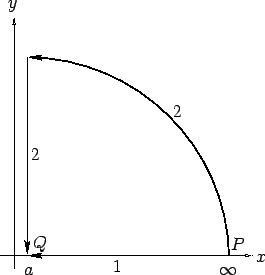Next: Surface Integrals Up: Vectors Previous: Vector Calculus
Richard Fitzpatrick 2007-07-14